# Samacheer Kalvi Books: Tamilnadu State Board Text Books Solutions

## Samacheer Kalvi 12th Computer Science Chapter 9 Lists, Tuples, Sets and Dictionary Notes PDF Download: Tamil Nadu STD 12th Computer Science Chapter 9 Lists, Tuples, Sets and Dictionary NotesSamacheer Kalvi 12th Computer Science Chapter 9 Lists, Tuples, Sets and Dictionary Notes PDF Download: Tamil Nadu STD 12th Computer Science Chapter 9 Lists, Tuples, Sets and Dictionary Notes

## Samacheer Kalvi 12th Computer Science Chapter 9 Lists, Tuples, Sets and Dictionary Notes PDF Download

We bring to you specially curated Samacheer Kalvi 12th Computer Science Chapter 9 Lists, Tuples, Sets and Dictionary Notes PDF which have been prepared by our subject experts after carefully following the trend of the exam in the last few years. The notes will not only serve for revision purposes, but also will have several cuts and easy methods to go about a difficult problem.

 Board Tamilnadu Board Study Material Notes Class Samacheer Kalvi 12th Computer Science Subject 12th Computer Science Chapter Chapter 9 Lists, Tuples, Sets and Dictionary Format PDF Provider Samacheer Kalvi Books

## How to Download Samacheer Kalvi 12th Computer Science Chapter 9 Lists, Tuples, Sets and Dictionary Notes PDFs?

2. Click on the Samacheer Kalvi 12th Computer Science Notes PDF.
3. Look for your preferred subject.
4. Now download the Samacheer Kalvi 12th Computer Science Chapter 9 Lists, Tuples, Sets and Dictionary notes PDF.

## Download Samacheer Kalvi 12th Computer Science Chapter 9 Lists, Tuples, Sets and Dictionary Chapterwise Notes PDF

PART – 1

12th Computer Science Chapter 9 Book Back Answers Question 1.
Pick odd one in connection with collection data type?
(a) List
(b) Tuple
(c) Dictionary
(d) Loop
(d) Loop

12th Computer Science Samacheer Question 2.
Let list1=[2 ,4, 6, 8, 10], then print (List1[-2]) will result in ……………………….
(a) 10
(b) 8
(c) 4
(d) 6
(b) 8

Computer 9 Question 3.
Which of the following function is used to count the number of elements in a list?
(a) Count( )
(b) Find( )
(c) Len( )
(d) Index( )
(c) Len( )

9th Computer Science Book Back Answers Question 4.
If List=[10, 20, 30, 40, 50] then List=35 will result –
(a) [35, 10, 20, 30, 40, 50]
(b) [10, 20, 30, 40, 50, 35]
(c) [10, 20, 35, 40, 50]
(d) [10, 35, 30, 40, 50]
(c) [10, 20, 35, 40, 50]

Question 5.
If List=[17, 23, 41, 10] then List.append (32) will result –
(a) [32, 17, 23, 41, 10]
(b) [17, 23, 41, 10, 32]
(c) [10, 17, 23, 32, 41]
(d) [41, 32, 23, 17, 10]
(b) [17, 23, 41, 10, 32]

Question 6.
Which of the following Python function can be used to add more than one element within an existing list?
(a) append( )
(b) append_more( )
(c) extend( )
(d) more( )
(c) extend( )

Question 7.
What will be the result of the following Python code?
S = [x**2 for x in range(5)]
print(S)
(a) [0, 1, 2, 4, 5]
(b) [0, 1, 4, 9, 16]
(c) [0, 1, 4, 9, 16, 25]
(d) [1, 4, 9, 16, 25]
(b) [0, 1, 4, 9, 16]

Question 8.
What is the use of type( ) function in python?
(a) To create a Tuple
(b) To know the type of an element in tuple.
(c) To know the data type of python object.
(d) To create a list.
(c) To know the data type of python object.

Question 9.
Which of the following statement is not correct?
(a) A list is mutable
(b) A tuple is immutable.
(c) The append( ) function is used to add an element.
(d) The extend( ) function is used in tuple to add elements in a list.
(d) The extend( ) function is used in tuple to add elements in a list.

Question 10.
Let set A={3, 6, 9}, set B={1, 3, 9} What will be the result of the following snippet?
print (setA|setB)
(a) {3, 6, 9, 1, 3, 9}
(b) {3, 9}
(c) {1}
(d) {1, 3, 6, 9}
(d) {1, 3, 6, 9}

Question 11.
Which of the following set operation includes all the elements that are in two sets but not the one that are common to two sets?
(a) Symmetric difference
(b) Difference
(c) Intersection
(d) Union
(a) Symmetric difference

Question 12.
The keys in Python, dictionary is specified by …………………………
(a) =
(b) ;
(c) +
(d) :
(d) :

PART – II

Question 1.
What is List in Python?

• A list in Python is known as a “sequence data type” like strings.
• It is an ordered collection of values enclosed within square brackets [ ].
• Each value of a list is called an element. It can be of any type such as numbers, characters, strings, and even nested lists as well.
• The list is constructed by placing expressions within square brackets separated by commas Any way of building two values to gather into one can be considered as a pair.
• Lists are a common method to do so. Therefore list can be called Pairs.

Question 2.
How will you access the list elements in reverse order?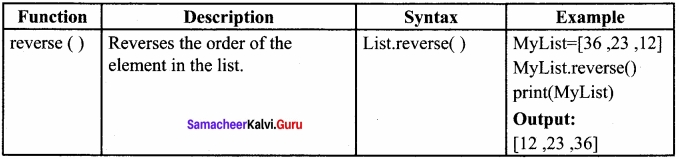Question 3.
What will be the value of x in the following python code?
List1 = [2,4,6, [1,3,5]] x = len(Listl)
Output:
x=4

Question 4.
Differentiate del with remove( ) function of List?
There are two ways to delete an element from a list viz. del statement and remove( ) function, del statement is used to delete known elements whereas remove( ) function is used to delete elements of a list if its index is unknown. The del statement can also be used to delete the entire list.

Question 5.
Write the syntax of creating a Tuple with n number of elements?
Syntax:
# Empty tuple
Tuple_Name = ()
# Tuple with n number elements
Tuple_Name = (El, E2, E2 En)
# Elements of a tuple without parenthesis
Tuple_Name = E1, E2, E3 ….. En

Question 6.
What is set in Python?
In python, a set is another type of collection data type. A Set is a mutable and unordered collection of elements without duplicates. That means the elements within a set cannot be repeated. This feature used to include membership testing and eliminating duplicate elements.

PART – III

Question 1.
What are the advantages of Tuples over a list?

• The elements of a list are changeable (mutable) whereas the elements of a tuple are unchangeable (immutable), this is the key difference between tuples and list.
• The elements of a list are enclosed within square brackets.
• The elements of a tuple are enclosed by parenthesis.
• Iterating tuples is faster than a list.

Question 2.
Write a short note about sort( )?
sort( ) function sorts the element in list.
Syntax:
List.sort (reverse = True/False, Key = myFunc)
Both arguments are optional

• If the reverse is set as True, list sorting is in descending order.
• Ascending is the default.
• Key=myFunc; “myFunc” – the name of the user defined function that specifies the sorting criteria.

Example
My List=[‘Thilothamma’, ’Tharani’, ‘Anitha’, ‘SaiSree’, ‘Lavanya’]
MyList.sort( )
print(MyList)
Output:
[‘Anitha’, ‘Lavanya’, ‘SaiSree’, ‘Tharani’, ‘Thilothamma’]

Question 3.
What will be the output of the following code?
list=[2**x for x in range(5)
print(list)
Output:
[1, 2, 4, 8, 16]

Question 4.
Explain the difference between del and clear( ) in dictionary with an example?
In Python dictionary, del keyword is used to delete a particular element. The clear( ) function is used to delete all the elements in a dictionary. To remove the dictionary, we can use del keyword with dictionary name.
Dict={‘Roll No’: 12001, ‘SName’: ‘Meena’, ‘Mark1’: 98, ‘Mar12’: 86}
print(“Dictionary elements before deletion: \n”, Dict)
del Dict[‘Mark1’] # Deleting a particular element
Dict.clear( ) # Deleting all elements

Question 5.
List out the set operations supported by python?
(i) Union:
It includes all elements from two or more sets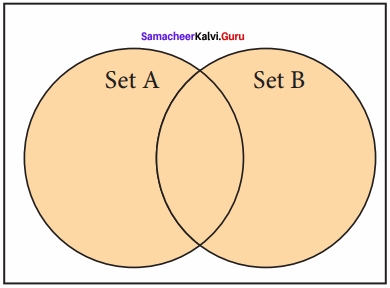In python, the operator | is used to the union of two sets. The function union( ) is also used to join two sets in python.

(ii) Intersection:
It includes the common elements in two sets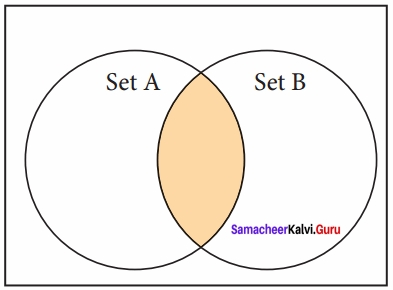The operator & is used to intersect two sets in python. The function intersection( ) is also used to intersect two sets in python.

(iii) Difference:
It includes all elements that are in the first set (say set A) but not in the second set (say set B)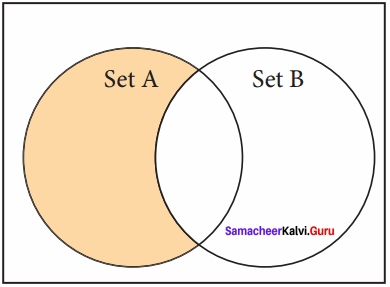The minus(-) operator is used to difference set operation in python. The function difference( ) is also used to difference operation.

(iv) Symmetric difference:
It includes all the elements that are in two sets (say sets A and B) but not the one that are common to two sets.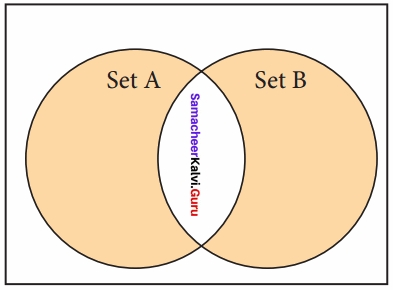The caret (^) operator is used to symmetric difference set operation in python. The function symmetric_difference( ) is also used to do the same operation.

Question 6.
What are the differences between List and Dictionary?
Difference between List and Dictionary

1. List is an ordered set of elements. But, a dictionary is a data structure that is used for matching one element (Key) with another (Value).
2. The index values can be used to access a particular element. But, in the dictionary key represents the index. Remember that, key may be a number of a string.
3. Lists are used to look up a value whereas a dictionary is used to take one value and look up another value.

PART – IV

Question 1.
What the different ways to insert an element in a list. Explain with suitable example. Inserting elements in a list?
The different ways to insert an element in a list are

1. Using append() function
2. Using extend() function
3. Using insert() function

1. append() function:
append() function is used to add a single element — ——— — ( 92 J*

Syntax:

Example:
>>>My list= [34,45,48]
>>>Mylist.append (90)
>>> print (MYlist)
[34,45,48,90]

• In the above example, Mylist is created with three elements.
• Through >>>Mylist.apperid(90) statement, an additional value 90 is included with the existing list as last element,

2. extend() function:
extend() function is used to add more than one element to an existing list.

Syntax:

Example:
In extend () function, multiple elements should be specified within square bracket as arguments of the function.
>>>Mylist= [34,45,48]
>>>Mylist.extend([71, 32, 29])
>>>print(Mylist)
[34,45,48, 90, 71, 32,29]

In the above code, extend( ) function is used to include multiple elements, the print statement shows all the elements of the list after the inclusion of additional elements.

3. insert () function:

• Append () function in Python is used to add more elements in a list. But, it includes elements at the end of a list.
• To include an element at your desired position, use the insert () function. The insert() function is used to insert an element at any position of a list.
• Syntax:
List, insert (position index, element)

Example:
>>>MyList= [34,98,47 .Kannan7, ‘Gowrisankar7, ‘Lenin7, ‘Sreenivasan7]
>>>print(MyList)
[34, 98,47, ‘Karman7, ‘Gowrisankar7, ‘Lenin7, ‘Sreenivasan’]
>>>MyList.insert(3, ‘Rarnakrishnan’)
>>>print(MyList)
[34,98,47, ‘Rarnakrishnan7, ‘Karman7, ‘Gowrisankar7, ‘Lenin7, ‘Sreenivasan’]

Question 2.
What is the purpose of range( )? Explain with an example?

• The range( ) is a function used to generate a series of values in Python.
• Using range () function, you can create a list with series of values.
• The range( ) function has three arguments.

Syntax:
range (start value, end value, step value)
where,

• start value – beginning value of series.
• Zero is the default beginning value. Z end value – the upper limit of series.
• Python takes the ending value as upper limit -1.
• step value – It is an optional argument, which is used to generate different intervals of values.

Example:
Generating odd numbers in between 1 and 10:
for x in range (1,11,2):
print(x)
Output:
1
3
5
7
9

Creating a list with series of values:

• Using the range() function, a list can be created with series of values.
• To convert the result of range () function into list, one more function called list is needed().
• The list() function makes the result of range() as a list.

Syntax:
List Variable = list (range ())

Example 1:
>>>Even_List = list(range(2,ll,2))
>>>print(EvenJList)
[2,4,6,8,10]

• In the above code, list() function takes the result of range () as Even_List elements.
• Thus, the Even_List list has the elements of the first five even numbers.
• Similarly, we can create any series of values using range() function.

Example 2:
Creating a list with squares of the first 10 natural numbers:
squares = [ ]
for x in range(l,ll):
s= x **2
squares, append (s)
print (squares)

Question 3.
What is a nested tuple? Explain with an example.?
In Python, a tuple can be defined inside another tuple; called a Nested tuple.
In a nested tuple, each tuple is considered as an element.
The for loop will be useful to access all the elements in a nested tuple.
Example:
Toppers = ((“Vinodini”, “XII-F”, 98.7), (“Soundarya”, “XII-H”, 97.5),
(“Tharani”, “XII-F”, 95.3), (“Saisri”, “XII-G”, 93.8))
for i in Toppers:
print(i)
Output:
(‘Vinodini’, ‘XII-F’, 98.7)
(‘Soundarya’, ‘XII-H’, 97.5)
(‘Tharani’, ‘XII-F’, 95.3)
(‘Saisri’, ‘XII-G’, 93.8)

Question 4.
Explain the different set operations supported by python with suitable example?
Set Operations:
As you learned in mathematics, python has also supported the set operations such as Union, Intersection, difference and Symmetric difference.
(i) Union:
It includes all elements from two or more sets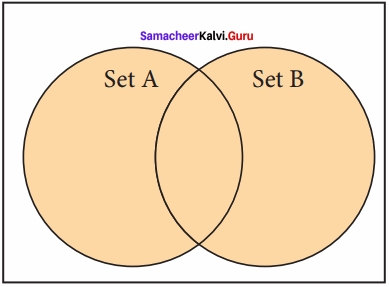In python, the operator | is used to union of two sets. The function union( ) is also used to join two sets in python.
Example: Program to Join (Union) two sets using union operator
set_A={2,4,6,8}
set_B={‘A’, ’B’, ‘C, ‘D’}
U_set=set_A|set_B
print(U_set)
Output:
{’D’, 2, 4, 6, 8, ‘B’, ’C, A’}

(ii) Intersection:
It includes the common elements in two sets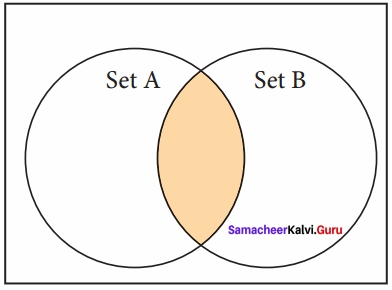The operator & is used to intersect two sets in python. The function intersection ) is also used to intersect two sets in python.
Example: Program to insect two sets using intersection operator
set_A={A’, 2, 4, ‘D’}
set_B={A’, ‘B’, ‘C’, ‘D’}
print(set_A & set_B)
Output:
{’A, ‘D’}
Example: Program to insect two sets using intersection operator
set_A={‘A’, 2, 4, ’D’}
set_B={A’, ‘B’, ‘C’, ‘D’}
print(set_A.intersection(set_B))
{‘A’, ‘D’}

(iii) Difference:
It includes all elements that are in first set (say set A) but not in the second set (say set B)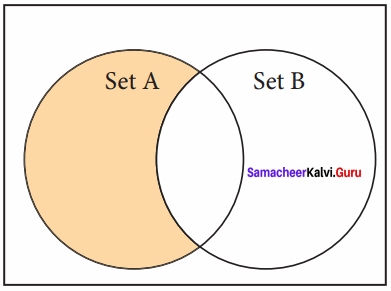The minus (-) operator is used to difference set operation in python. The function difference() is also used to difference operation.
Example: Program to difference of two sets using minus operator
set_A={‘A’, 2, 4, ‘D’} set_B={‘A’, ‘B’, ‘C, ‘D’} print(set_A – set_B)
Output:
{2,4}
Example: Program to difference of two sets using difference function
set_A={‘A’, 2, 4, TV} set_B={‘A’, ’B’, ’C, ’D’} print(set_A.difference(set_B))
Output:
{2,4}

(iv) Symmetric difference:
It includes all the elements that are in two sets (say sets A and B) but not the one that are common to two sets.The caret (^) operator is used to symmetric difference set operation in python. The function symmetric_difference( ) is also used to do the same operation.
Example: Program to symmetric difference of two sets using caret operator
set_A={‘A’, 2, 4, ‘D’}
set_B={‘A’, ‘B’, ‘C, ‘D’}
print(set_A^A set_B)
Output:
{2, 4, ‘B’, ‘C’}
Example: Program to difference of two sets using symmetric difference function
set_A={‘A’, 2, 4, ‘D’}
set_B={‘A’, ‘B’, ‘C’, ‘D’}
print(set_A. symmetric_difference(set_B))
Output:
{2, 4, ‘B’, ‘C’}

Practice Programs

Question 1.
Write a program to remove duplicates from a list.
Method I:
mylist = [2,4,6,8,8,4,10]
myset = set(mylist)
print(myset)
Output:
{2, 4, 6, 8, 10}
Method II:
def remove(duplicate):
final_list=[ ]
for num in duplicate:
if num not in final_list:
final_list.append(num)
return final_list
duplicate = [2, 4, 10, 20, 5, 2, 20, 4]
print(remove(duplicate))
Output:
[2, 4, 10, 20, 5]

Question 2.
Write a program that prints the maximum value in a Tuple?
tuple = (456, 700, 200)
print(“max value : “, max(tuple))
Output:
max value : 700

Question 3.
Write a program that finds the sum of all the numbers in a Tuples using while loop?
tuple = (1, 5, 12)
s = 0
i = 0
while(i < len (tuple)):
s = s + tuple[i]
i+ = 1
print(“Sum of elements in tuple is “, s)
Output:
Sum of elements in tuple is 18

Question 4.
Write a program that finds sum of all even numbers in a list?
numlist = [ ]
evensum = 0
number = int(input(“Please enter the total no of list elements”))
for i in range(1, number +1):
value = int(input(“Please enter the value “))
numlist.append(value)
for j in range(number):
if(numlist[j]% 2 == 0):
even_sum = even_sum + numlist[j]
print(“Sum of even no. in this list = “, even_sum)
Output:
Please enter the total no of list elements : 5
Please enter the value : 10
Please enter the value : 11
Please enter the value : 12
Please enter the value : 13
Please enter the value : 14
The sum of even no. in this list = 60

Question 5.
Write a program that reverse a list using a loop?
def reverse(list):
list.reverse( )
return list
list = [10, 11, 12, 13, 14, 15]
print(reverse(list))
Output:
15, 14, 13, 12, 11, 10

Question 6.
Write a program to insert a value in a list at the specified location?
vowel = [‘a’, ‘e’, ‘i’, ‘u’]
vowel.insert(3, ‘o’)
print(‘updated list’, vowel)
Output:
updated list [‘a’, ‘e’, ‘i’, ‘o’, ‘u’]

Question 7.
Write a program that creates a list of numbers from 1 to 50 that are either divisible by 3 or divisible by 6?
n = [ ]
s = [ ]
for x in range(1, 51):
n.append(x)
for x in range(1, 51):
if(x%3 == 0) or (x % 6 == 0):
s.append(x)
print(“The numbers divisible by 3 or 6 is “, s)
Output:
The numbers divisible by 3 or 6 is
[3, 6, 9, 12, 15, 18, 21, 24, 27, 30, 33, 36, 39, 42, 45, 48]

Question 8.
Write a program to create a list of numbers in the range 1 to 20. Then delete all the numbers from the list that are divisible by 3?
num = [ ]
for x in range(1, 21):
num.append(x)
print(“The list of numbers from 1 to 20 =”, num)
for index, i in enumerate(num):
if(i % 3 == 0)
del num[index]
print(“The list after deleting numbers”, num)
Output:
The list of numbers from 1 to 20 = [1,2,3,4… 20]
The list after deleting numbers[1, 2, 4, 5, 7, 8, 10, 11, 13, 14, 16, 17, 19, 20]

Question 9.
Write a program that counts the number of times a value appears in the list. Use a loop to do the same?
a = [ ]
n = int(input”Enter number of elements :”))
for i in range(l, n+1):
b = int(input(“Enter element”))
a.append(b)
k = 0
num = int(input(“Enter the number to be counted : “))
for j in a:
if(j == num):
k = k+1
print(“Number of times”, num, “appears is”, k)
Output:
Enter number of elements : 4
Enter element: 23
Enter element: 45
Enter element: 23
Enter element: 67
Enter the number to be counted : 23
Number of times 23 appears is 2

Question 10.
Write a program that prints the maximum and minimum value in a dictionary?
my_dict = {‘x’: 500, ‘y’: 5874, ‘z’: 560}
val = my_dict.values( )
print(‘max value’, max(val))
print(‘min value’, min(val))
Output:
max value 5874
min value 500

### Samacheer Kalvi 12th Computer Science Lists, Tuples, Sets and Dictionary Additional Questions and Answers

PART – 1

Question 1.
Which of the following is not a data type in Python?
a) List
b) Tuples
c) String
c) Set
c) String

Question 2.
A ………………………… is a sequence data type like strings.
(a) List
(b) Tuples
(c) Set
(d) Dictionary
(a) List

Question 3.
Which of the following data type enclosed with [ ]?
a) Tuples
b) List
c) Dictionary
d) Set
b) List

Question 4.
The position of an element is indexed with numbers beginning with …………………………….
(a) n
(b) n-1
(c) 0
(d) 1
(c) 0

Question 5.
(1) mylist[ ] – (i) tuple
(2) mylist[10,[2,4,6]] – (ii) Empty tuple
(3) t=(23,56,89) – (iii) Nested list
(4) lis=( ) – (iv) empty list
(a) 1-(iv), 2-(iii), 3-(i), 4-(ii)
(b) 1-(i), 2-(ii), 3-(iii), 4-(iv)
(c) 1-(iv), 2-(ii), 3-(i), 4-(iii)
(d) 1-(i), 2-(iii), 3-(iv), 4-(ii)
(a) 1-(iv), 2-(iii), 3-(i), 4-(ii)

Question 6.
………………………… is used to access an element in a list
(a) element
(b) i
(c) index
(d) tuple
(c) index

Question 7.
Index value can be positive or negative in the list. (True / False)
True

Question 8.
Sim = [‘S’, 2,3, [4,5,6]] is an example of
a) Tuple
b) Set
c) List
d) Dictionary
c) List

Question 9.
………………………. are used to access all elements from a list.
(a) If
(b) loop
(c) array
(d) tuple
(b) loop

Question 10.
Find the Output:
marks = [10, 23, 41, 75]
i = -1
while i >= – 4:
print(marks[i])
i = i + – 1
(a) 1 2 3 4
(b) 10, 23, 41, 75
(c) 75, 41, 23, 10
(d) 0, 41, 23, 0
(c) 75, 41, 23, 10

Question 11.
Which of the following operator can be used to alter the range of elements in the list?
a) = =
b) ::
c) = .
d) :
c) =

Question 12.
In changing list elements, …………………………. is the upper limit of this range.
(a) Index from
(b) Index to
(c) Index with
(d) Index
(b) Index to

Question 13.
If the range is specified as [1 : 5], it will update the elements from …………………………..
(a) 2 to 4
(b) 1 to 5
(c) 1 to 4
(d) 2 to 5
(c) 1 to 4

Question 14.
……………………….. function is used to add a single element to the list.
append( )

Question 15.
Which function can also be used to delete an element using the given index value.
a) erase
b) pop
c) delete ()
d) push()
b) pop

Question 16.
list = [34, 45, 48]
list.extend([71, 32, 29]) results in ………………………….
[35, 45, 48, 71, 32, 29]

Question 17.
………………………… function is used to insert an element at any position of a list.
insert( )

Question 18.
Find the correct statement from the following
(a) when a new element is inserted into the list, the existing elements shift one position to the right
(b) when a new element is inserted in the list, the existing element shifts one position to the left.
(a) when a new element is inserted into the list, the existing elements shift one position to the right

Question 19.
How many ways of deleting the elements from a list are there?
(a) 1
(b) 2
(c) 3
(d) 4
(b) 2

Question 20.
The two ways of deleting elements from a list are ……………………….. and …………………………
del and remove( )

Question 21.
Which function is used to delete elements of a list if its index is unknown?
(a) del
(b) delete
(c) remove( )
(d) backspace
(c) remove( )

Question 22.
Which statement is used to delete known elements?
(a) del
(b) delete
(c) remove
(d) rem
(a) del

Question 23.
………………………… statement deletes the entire list.
del

Question 24.
…………………….. function deletes the element using the given index value.
pop( )

Question 25.
When you try to print the list which is already cleared, ……………………….. is display without any elements
[ ] or empty square bracket

Question 26.
…………………………… function is used to generate a series of values in python
(a) range
(b) series
(c) Fill series
(d) Autofill
(a) range

Question 27.
The range( ) function has ………………………. arguments.
(a) 1
(b) 2
(c) 3
(d) 4
(c) 3

Question 28.
Which is an optional argument in range( ) function …………………………..
(a) start value
(b) end value
(c) step value
(d) default
(c) step value

Question 29.
The ……………………… function is used to create a list in python
list( )

Question 30.
……………………… is the simplest way of creating a sequence of elements that satisfy certain conditions returns a copy of the list.
List comprehension

Question 31.
……………….. returns a copy of the list
copy( )

Question 32.
x = mylist = [36, 12, 12]
x = mylist.count(12)
print(x) gives the vlaue as
(a) 2
(b) 3
(c) 0
(d) 1
(a) 2

Question 33.
………………….. returns the index value of the first recurring element.
index( )

Question 34.
How many arguments are there in the sort( ) function?
(a) 1
(b) 2
(c) 3
(d) 4
(b) 2

Question 35.
……………………… consists of a number of values separated by a comma and enclosed within parenthesis
(a) list
(b) tuples
(c) dictionary
(d) sets
(b) tuples

Question 36.
The term ………………………. in Latin represents an abstraction of the sequence of numbers.
(a) list
(b) tuples
(c) set
(d) dictionary
(b) tuples

Question 37.
Identify the wrong statement from the following:
(a) The elements of the tuple are enclosed by parenthesis.
(b) The elements of a tuple can be even defined without parenthesis
(c) The list elements have to be given in square brackets
(d) Iterating list is faster than tuples.
(d) Iterating list is faster than tuples.

Question 38.
The …………………….. function is used to create tuples from a list.
tuple( )

Question 39.
Creating a tuple with one element is called ……………………….. tuple.
Singleton

Question 40.
Find the wrong tuple.
(a) mytup = (10)
(b) mytup = (10)
(c) print(tup[: ])
(d) tup(10, 20)
(d) tup(10, 20)

Question 41.
To delete an entire tuple, …………………….. command is used.
(a) del
(b) delete
(c) clear
(d) remove
(a) del

Question 42.
Which operator is used to join two tuples?
(a) –
(b) _
(c) +
(d) +:
(c) +

Question 43.
…………………….. assignment is a powerful feature in python.
Tuple

Question 44.
(x, y) = (3**2, 15%2)
(a) 6 1
(b) 6 7
(c) 9 1
(d) 9 7
(c) 9 1

Question 45.
Which one of the following is the tuple assignment operator?
(a) +=
(b) =
(c) ==
(d) *=
(b) =

Question 46.
How many values can be returned by the functions in python?
(a) 1
(b) 2
(c) 3
(d) many
(d) many

Question 47.
A tuple defined in another tuple is called as ……………………….
Nested tuple

Question 48.
…………………………. feature is used to include membership testing and eliminate duplicate elements.
Set

Question 49.
A …………………………… is a mutable and unordered collection of elements without duplicates.
Set

Question 50.
Which is true related to sets?
(a) mutable
(b) unordered
(c) No duplicates
(d) All are true
(d) All are true

Question 51.
A list of tuples can be converted as set by using …………………………. function?
(a) set
(b) create set
(c) change
(d) alter
(a) set

Question 52.
Which operator joins two sets?
(a) +
(b) |
(c) ||
(d) &
(b) |

Question 53.
Join is called as ……………………… in sets
(a) union
(b) intersection
(c) difference
(d) symmetric difference
(a) union

Question 54.
Identify the intersection operator.
(a) +
(b) –
(c) .
(d) &
(d) &

Question 55.
Which operator is used to do a difference in the set?
(a) +
(b) –
(c) :
(d) &
(b) –

Question 56.
Which is the symmetric difference operator?
(a) +
(b) –
(c) ^
(d) &
(c) ^

Question 57.
………………………. is used to separate the elements in the dictionary
Comma

Question 58.
The key-value pairs are enclosed with ……………………………
(a) <>
(b) [ ]
(c) { }
(d) ( )
(c) { }

Question 59.
The mixed collection of elements are called………………………….
(a) list
(b) tuples
(c) sets
(d) dictionary
(d) dictionary

Question 60.
Identify the correct statement.
(a) The dictionary type stores an index along with its element
(b) The dictionary type stores a key along with its element
(a) The dictionary type stores an index along with its element

Question 61.
Which part is optional in dictionary comprehension?
(a) If
(b) expression
(c) var
(d) sequences
(a) If

Question 62.
Find the statement which is wrong. When you assign a value to the key
(a) it will be appended
(b) it will overwrite the old data
(a) it will be appended

Question 63.
Pick an odd one with including elements in the list.
(a) append( )
(b) extend( )
(c) insert( )
(d) include
(d) include

Question 64.
Pick the odd one with deleting elements from a list.
(a) del
(b) remove( )
(c) pop( )
(d) clear
(d) clear

PART – II

Question 1.
Explain the “Singleton” tuple.

• While creating a tuple with a single element, add a comma at the end of the element.
• In the absence of a comma, Python will consider the element as an ordinary data type; not a tuple.
• Creating a Tuple with one element is called “Singleton” tuple.

Example:
>>> MyTup4 = (10)
>>> type(MyTup4)
< class’int’>
>>> MyTup5 = (10,)
>>> type(MyTup5)
< class ‘tuple’ >

Question 2.
Fill the table
marks = [10, 23, 41, 75]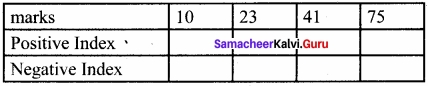Example
Marks [10, 23, 41, 75]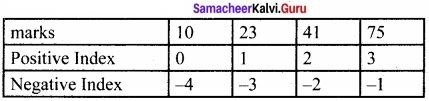Question 3.
Give the syntax to access an element from a list?
To access an element from a list, write the name of the list, followed by the index of the elements enclosed within square brackets.
Syntax:
List_Variable = [El, E2, E3 …………….. En]
print (List_Variable [index of a element])

Question 4.
How to delete an entire tuple? Give an example.
To delete an entire tuple, the del command can be used.
Syntax:
del tuple_name

Example:
Tupl = (2,4,6,8,10)
print(“The elements of Tupl is “, Tup 1)
del Tupl
print (Tup 1)

Question 5.
Give the syntax for changing list elements?
Syntax:
List_Variable [index of an element] = Value to be changed
List_Variable [index from : index to] = Values to changed
Where, index from is the beginning index of the range; index to is the upper limit of the range which is excluded in the range.

Question 6.
Give the syntax for append, extend and insert?
Syntax:
List, extend ( [elements to be added])
List, insert (position index, element)

Question 7.
What is a nested list? Give example.
A nested list is a list containing another list as an element.
Example:
Mylist = [ “Welcome’, 3.14,10, [2,4,6] ]
In the above example, Mylist contains another list [2,4,6] as an element.

Question 8.
How will you create a list with series of value?
Using the range( ) function, you can create a list with series of values. To convert the result of range( ) function into list, we need one more function called list( ). The list( ) function makes the result of range( ) as a list.
Syntax:
List_Varibale = list (range ( ))

Question 9.
Write note on list comprehensions?
List comprehensions:
List comprehension is the simplest way of creating a sequence of elements that satisfy a certain condition.
Syntax:
List = [expression for variable in range]

Question 10.
Define singleton tuple?
While creating a tuple with a single element, add a comma at the end of the element. In the absence of a comma, Python will consider the element as an ordinary data type; not a tuple. Creating a Tuple with one element is called the “Singleton” tuple.
MyTup5 = (10,)

Question 11.
Write note on dictionary comprehensions?
In Python, comprehension is another way of creating a dictionary. The following is the syntax of creating such a dictionary.
Syntax
Diet = { expression for variable in sequence [if condition] }

PART – III

Question 1.
How will you create a list in python? Explain with syntax and examples?
In python, a list is simply created by using square bracket. The elements of list should be specified within square brackets. The following syntax explains the creation of list.
Syntax:
Variable = [element – 1, element – 2, element – 3 element – n]
Example:
Marks = [10, 23, 41, 75]

Question 2.
How will you find the length of the list. Give example?
The len( ) function in Python is used to find the length of a list, (i.e., the number of elements in a list). Usually, the len( ) function is used to set the upper limit in a loop to read all the elements of a list. If a list contains another list as an element, len( ) returns that inner list as a single element.
Example: Accessing single element
>>> MySubject = [“Tamil”, “English”, “Comp. Science”, “Maths”]
>>> len(MySubject)
4

Question 3.
Give the 3 different syntax formats for deleting the elements from a list?
Syntax:
del List [index of an element]
# to delete a particular element del List [index from : index to]
# to delete multiple elements
del List
# to delete entire list

Question 4.
Give the syntax for remove, pop and clear?
Syntax:
List.remove(element) # to delete a particular element
List.pop(index of an element)
List, clear( )

Question 5.
Write a program that creates a list of numbers from 1 to 20 that are divisible by 4. Program to create a list of numbers from 1 to 20 that are divisible by 4
divBy4=[ ]
for i in range(21):
if (i%4= =0):
divBy4.append(i)
print(divBy4)
Output
[0, 4, 8, 12, 16, 20]

Question 6.
Write a program to join two tuple assignment?
# Program to join two tuples
Tup 1 = (2,4,6,8,10)
Tup2 = (1,3,5,7,9)
Tup3 = Tup1 + Tup2
print(Tup3)
Output
(2,4,6,8,10,1,3,5,7,9)

Question 7.
Write note on tuple assignment?
Tuple assignment is a powerful feature in Python. It allows a tuple variable on the left of the assignment operator to be assigned to the values on the right side of the assignment operator.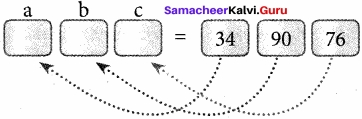Example
>>> (a, b, c) = (34, 90, 76)
>>> print(a,b,c)
34 90 76
# expression are evaluated before assignment
>>> (x, y, z, p) = (2**2, 5/3+4, 15%2, 34>65)
>>> print(x,y,z,p)
4 5.666666666666667 1 False

Question 8.
How will you create a set in python?
A set is created by placing all the elements separated by comma within a pair of curly brackets.
The set( ) function can also used to create sets in Python.
Syntax:
Set Variable = {El, E2, E3 ………………. En}
Example:
>>> S1={1,2,3,’A’,3.14}
>>> print(S1)
{1,2, 3, 3.14, ‘A’}
>>> S2={1,2,2,’A’,3.14}
>>> print(S2)
{1,2,’A’, 3.14}

Question 9.
Write note on dictionaries. Give syntax?
A dictionary is a mixed collection of elements. The dictionary type stores a key along with its element. The keys in a Python dictionary is separated by a colon ( : ) while the commas work as a separator for the elements. The key value pairs are enclosed with curly braces { }. Syntax of defining a dictionary:
Dictionary_Name =
{ Key_l: Value_1,
Key_2: Value_2,
……………..
Key_n: Value_n
}

PART – IV

Question 1.
Explain Various Functions in list?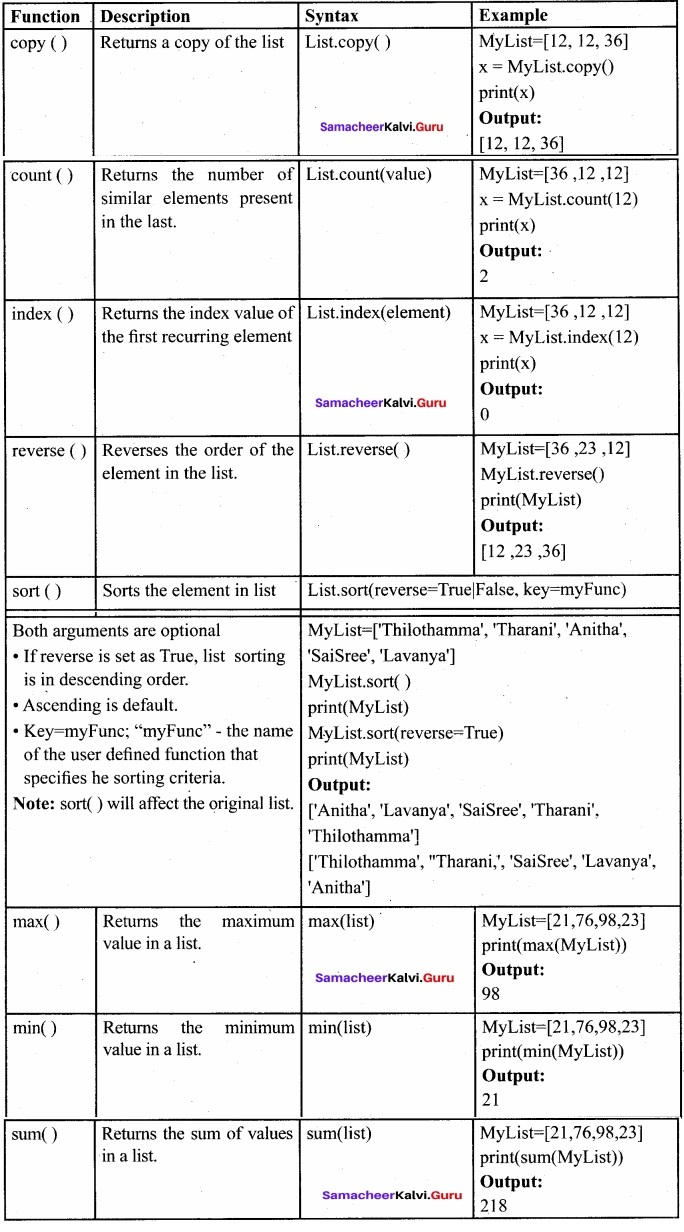Question 2.
Explain how to access all elements of a list using for loop with a suitable example.

• In python, ‘for loop’ is used to access all the elements in a list one by one.
• This is just like the for a keyword in other programming languages such as C++.

Syntax:
for index_var in list:
print (index_var)

Here, index_var represents the index value of each element in the list. Python reads this “for” statement like English: “For (every) element in (the list of) list and print (the name of the) list items”

Example: Marks=[23,45, 67, 78,98]
for x in Marks:
print(x)
Output:
23
45
67
78
98

• In the above example, the Marks list has 5 elements; each element is indexed from 0 to 4.
• The Python reads the for loop and print statements like English: “For (every) element (represented as x) in (the list of) Marks and print (the values of the) elements’.

Question 3.
Write a program using list to generate the Fibonacci series and find sum. Program to generate in the Fibonacci series and store it in a list. Then find the sum of all values?
a=-1
b=1
n=int(input(“Enter no. of terms: “))
i=0
sum=0
Fibo=[ ]
while i<n:
s = a + b
Fibo.append(s)
sum+=s
a = b
b = s
i+=1
print(“Fibonacci series upto “+ str(n) +” terms is : ” + str(Fibo))
print(“The sum of Fibonacci series: “,sum)
Output
Enter no. of terms: 10
Fibonacci series upto 10 terms is : [0, 1, 1, 2, 3, 5, 8, 13, 21, 34]
The sum of Fibonacci series: 88

Question 4.
Write a program to swap two values using tuple assignment
Program to swap two values using tuple assignment
a = int(input(“Enter value of A: “))
b = int(input(“Enter value of B: “))
print(“Value of A = “, a, “\n Value of B = “, b)
(a, b) = (b, a)
print(“Value of A = “, a, “\n Value of B = “, b)
Output:
Enter value of A: 54
Enter value of B: 38
Value of A = 54
Value of B = 38
Value of A = 38
Value of B = 54

Question 5.
Consider the following tuple declaration:
>>> Mytyple=tuple([x**2 for x in range(2,11,2)])
What will be the output of the following print statements?
(i) >>> print(Mytuple[2:3])
(ii) >>> print(Mytuple[3:1])
(iii) >>> print(Mytuple[:])
Output:
>>> Mytuple=tuple([x**2 for x in range(2,ll,2)])
>>> print(Mytuple[2:3])
(36,)
>>> print(Mytuple[3:])
(64,100)
>>> print(Mytuple[:])
(4,16, 36,64,100)

## How to Prepare using Samacheer Kalvi 12th Computer Science Chapter 9 Lists, Tuples, Sets and Dictionary Notes PDF?

Students must prepare for the upcoming exams from Samacheer Kalvi 12th Computer Science Chapter 9 Lists, Tuples, Sets and Dictionary Notes PDF by following certain essential steps which are provided below.

• Use Samacheer Kalvi 12th Computer Science Chapter 9 Lists, Tuples, Sets and Dictionary notes by paying attention to facts and ideas.
• Pay attention to the important topics
• Refer TN Board books as well as the books recommended.
• Correctly follow the notes to reduce the number of questions being answered in the exam incorrectly
• Highlight and explain the concepts in details.

## Frequently Asked Questions on Samacheer Kalvi 12th Computer Science Chapter 9 Lists, Tuples, Sets and Dictionary Notes

#### How to use Samacheer Kalvi 12th Computer Science Chapter 9 Lists, Tuples, Sets and Dictionary Notes for preparation??

Read TN Board thoroughly, make separate notes for points you forget, formulae, reactions, diagrams. Highlight important points in the book itself and make use of the space provided in the margin to jot down other important points on the same topic from different sources.

#### How to make notes for Samacheer Kalvi 12th Computer Science Chapter 9 Lists, Tuples, Sets and Dictionary exam?

Read from hand-made notes prepared after understanding concepts, refrain from replicating from the textbook. Use highlighters for important points. Revise from these notes regularly and formulate your own tricks, shortcuts and mnemonics, mappings etc.
Share: